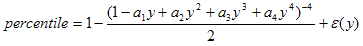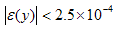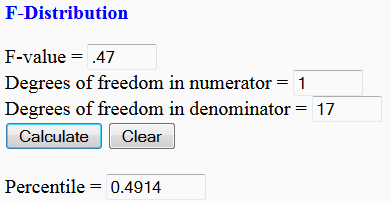# F-Distribution Table - use this calculator instead!

This tool is an equivalent of an F-distribution table and calculates percentile values for given values on an F-distribution curve. You must provide the F-value, and the degrees of freedom in both numerator and  denominator. The F-distribution calc makes it very easy to find the cumulative probability associated with an F-value.F-distribution curve

The F-distribution table is approximated using the following formula:where

a1 = 0.196854
a2 = 0.115194
a3 = 0.000344
a4 = 0.019527d1 = degrees of freedom in numerator
d2 = degrees of freedom in denominator### Example:

What is the percentile on a F-distribution table when the F-value is 2 and the degrees of freedom are both 5?

We use our online table and enter:

F-value = 2
Degrees of freedom in numerator = 5
Degrees of freedom in denominator = 5

The result is

Percentile =     0.7681

### Another example:

If the f-value is 0.47, the degrees of freedom in the numerator is just 1 and in the denominator is 17, use the tool like this, and get:Try it!!

 Please, enable JavaScript codes on your navigator, so you can use this calculator.

 F-Distribution Calculator F-value = Degrees of freedom in numerator = Degrees of freedom in denominator = Percentile =

From 'F-Distribution Table' to home

From 'F-Distribution Table' to Free Online Calculators